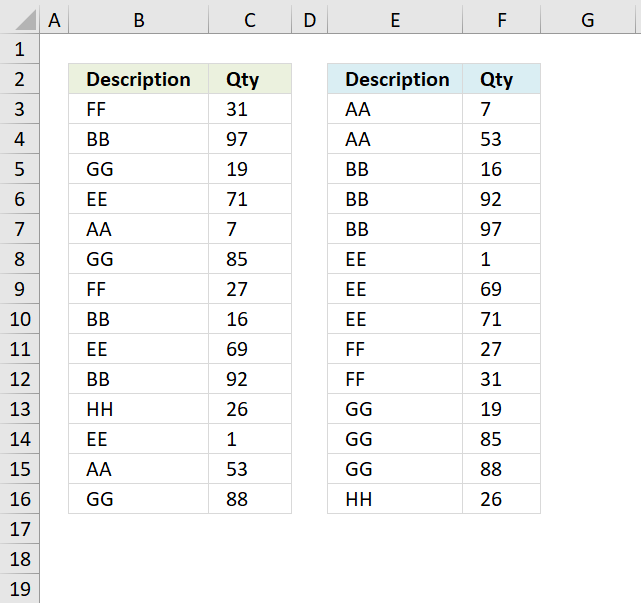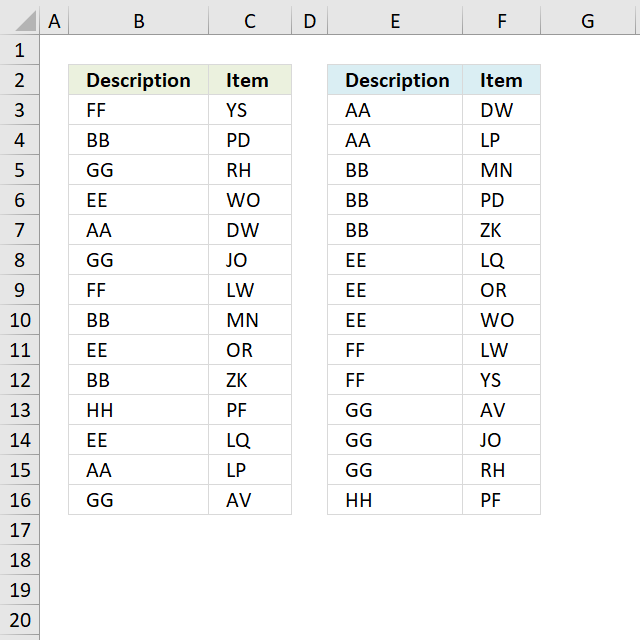Author: Oscar Cronquist Article last updated on December 12, 2018The image above shows a table with two columns in cell range B3:C16, it contains random text values in column B and random numbers in column C. The array formula in cell E3 sorts the text values in column B from A to Z, the array formula in cell F3 sorts the numbers in column C based on the adjacent value in column E.

In other words, the records are sorted based on the text value and then on numbers.

Array formula in E3:

=INDEX(\$B\$3:\$B\$16, MATCH(SMALL(COUNTIF(\$B\$3:\$B\$16, "<"&\$B\$3:\$B\$16), ROWS(\$A\$1:A1)), COUNTIF(\$B\$3:\$B\$16, "<"&\$B\$3:\$B\$16), 0))

To enter an array formula, type the formula in a cell then press and hold CTRL + SHIFT simultaneously, now press Enter once. Release all keys.

The formula bar now shows the formula with a beginning and ending curly bracket telling you that you entered the formula successfully. Don't enter the curly brackets yourself.

Array formula in F3:

=SMALL(IF(E3=\$B\$3:\$B\$16,\$C\$3:\$C\$16,""),COUNTIF(\$E\$3:E3,E3))

### Explaining formula in cell E3

#### Step 1 - Create an array of numbers representing the rank order if the list were sorted

The COUNTIF function counts values based on a condition or criteria, in this case, we use the < less than sign to compare the value against all other values.

COUNTIF(\$B\$3:\$B\$16, "<"&\$B\$3:\$B\$16)

becomes

COUNTIF({"FF"; "BB"; "GG"; "EE"; "AA"; "GG"; "FF"; "BB"; "EE"; "BB"; "HH"; "EE"; "AA"; "GG"},{"<FF"; "<BB"; "<GG"; "<EE"; "<AA"; "<GG"; "<FF"; "<BB"; "<EE"; "<BB"; "<HH"; "<EE"; "<AA"; "<GG"})

and returns

{8; 2; 10; 5; 0; 10; 8; 2; 5; 2; 13; 5; 0; 10}.

#### Step 2 - Get the k-th smallest number from array

To be able to return a new value in a cell each I use the SMALL function to filter column numbers from smallest to largest.

The ROWS function keeps track of the numbers based on an expanding cell reference. It will expand as the formula is copied to the cells below.

SMALL(COUNTIF(\$B\$3:\$B\$16, "<"&\$B\$3:\$B\$16), ROWS(\$A\$1:A1))

becomes

SMALL({8; 2; 10; 5; 0; 10; 8; 2; 5; 2; 13; 5; 0; 10}, ROWS(\$A\$1:A1))

becomes

SMALL({8; 2; 10; 5; 0; 10; 8; 2; 5; 2; 13; 5; 0; 10}, 1)

and returns 0 (zero).

#### Step 3 - Find position of number in array

The MATCH function finds the relative position of a value in an array or cell range.

MATCH(SMALL(COUNTIF(\$B\$3:\$B\$16, "<"&\$B\$3:\$B\$16), ROWS(\$A\$1:A1)), COUNTIF(\$B\$3:\$B\$16, "<"&\$B\$3:\$B\$16), 0)

becomes

MATCH(0, COUNTIF(\$B\$3:\$B\$16, "<"&\$B\$3:\$B\$16), 0)

becomes

MATCH(0, {8; 2; 10; 5; 0; 10; 8; 2; 5; 2; 13; 5; 0; 10}, 0)

and returns 5.

#### Step 4 - Get value

The INDEX function returns a value based on row number (and column number if needed)

INDEX(\$B\$3:\$B\$16, MATCH(SMALL(COUNTIF(\$B\$3:\$B\$16, "<"&\$B\$3:\$B\$16), ROWS(\$A\$1:A1)), COUNTIF(\$B\$3:\$B\$16, "<"&\$B\$3:\$B\$16), 0))

becomes

INDEX(\$B\$3:\$B\$16, 5)

and returns "AA" in cell E3.The image above demonstrates a table containing text values in both columns, you need a different array formula to sort the second column now containing text values.

Array formula in E3:

=INDEX(\$B\$3:\$B\$16, MATCH(SMALL(COUNTIF(\$B\$3:\$B\$16, "<"&\$B\$3:\$B\$16), ROW(1:1)), COUNTIF(\$B\$3:\$B\$16, "<"&\$B\$3:\$B\$16), 0))

copied down as far as needed.

Array formula in F3:

=INDEX(\$C\$3:\$C\$16, MATCH(SMALL(COUNTIF(\$B\$3:\$B\$16, "<"&\$B\$3:\$B\$16)+(1/(COUNTIF(\$C\$3:\$C\$16, ">"&\$C\$3:\$C\$16)+1)), ROW(1:1)), COUNTIF(\$B\$3:\$B\$16, "<"&\$B\$3:\$B\$16)+(1/(COUNTIF(\$C\$3:\$C\$16, ">"&\$C\$3:\$C\$16)+1)), 0))

### Download Excel *.xlsx file

Sort two columns using an array formula.xlsx